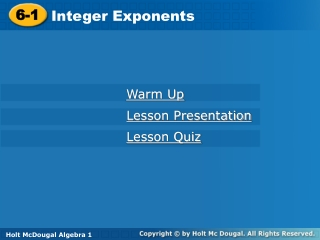DownloadDownload Presentation6-1

# 6-1

Télécharger la présentation## 6-1

- - - - - - - - - - - - - - - - - - - - - - - - - - - E N D - - - - - - - - - - - - - - - - - - - - - - - - - - -
##### Presentation Transcript

1. 6-1 Integer Exponents Warm Up Lesson Presentation Lesson Quiz Holt McDougal Algebra 1 Holt Algebra 1

2. Warm Up Evaluate each expression for the given values of the variables. 1.x3y2 for x = –1 and y = 10 2. for x = 4 and y = (–7) Write each number as a power of the given base. –100 3. 64; base 4 43 4. –27; base (–3) (–3)3

3. Objectives Evaluate expressions containing zero and integer exponents. Simplify expressions containing zero and integer exponents.

4.  5  5  5  5 You have seen positive exponents. Recall that to simplify 32, use 3 as a factor 2 times: 32 = 3  3 = 9. But what does it mean for an exponent to be negative or 0? You can use a table and look for a pattern to figure it out. 55 54 53 52 51 50 5–1 5–2 625 3125 125 25 5

5. When the exponent decreases by one, the value of the power is divided by 5. Continue the pattern of dividing by 5.

6. Remember! Base x Exponent 4

7. Notice the phrase “nonzero number” in the previous table. This is because 00 and 0 raised to a negative power are both undefined. For example, if you use the pattern given above the table with a base of 0 instead of 5, you would get 0º = . Also 0–6 would be = . Since division by 0 is undefined, neither value exists.

8. Reading Math 2–4 is read “2 to the negative fourth power.”

9. gal is equal to Example 1: Application One cup is 2–4 gallons. Simplify this expression.

10. 5-3 m is equal to Check It Out! Example 1 A sand fly may have a wingspan up to 5–3 m. Simplify this expression.

11. Example 2: Zero and Negative Exponents Simplify. A. 4–3 B. 70 Any nonzero number raised to the zero power is 1. 7º = 1 C. (–5)–4 D. –5–4

12. Caution In (–3)–4, the base is negative because the negative sign is inside the parentheses. In –3–4 the base (3) is positive.

13. Check It Out! Example 2 Simplify. a. 10–4 b. (–2)–4 c. (–2)–5 d. –2–5

14. Use the definition Example 3A: Evaluating Expressions with Zero and Negative Exponents Evaluate the expression for the given value of the variables. x–2 for x = 4 Substitute 4 for x.

15. Example 3B: Evaluating Expressions with Zero and Negative Exponents Simplify the expression for the given values of the variables. –2a0b-4 for a = 5 and b = –3 Substitute 5 for a and –3 for b. Evaluate expressions with exponents. Write the power in the denominator as a product. Simplify the denominator. Simplify.

16. Check It Out! Example 3a Evaluate the expression for the given value of the variable. p–3 for p = 4 Substitute 4 for p. Simplify exponent. Write the power in the denominator as a product. Simplify the denominator.

17. for a = –2 and b = 6 Check It Out! Example 3b Evaluate the expression for the given values of the variables. Substitute –2 for a and 6 for b. Simplify expressions with exponents. Write the power in the denominator as a product. Simplify the denominator. 2 Simplify.

18. What if you have an expression with a negative exponent in a denominator, such as ? or Definition of a negative exponent. Substitute –8 for n. Simplify the exponent on the right side. An expression that contains negative or zero exponents is not considered to be simplified. Expressions should be rewritten with only positive exponents. So if a base with a negative exponent is in a denominator, it is equivalent to the same base with the opposite (positive) exponent in the numerator.

19. B. Example 4: Simplifying Expressions with Zero and Negative Numbers Simplify. A. 7w–4

20. and Example 4: Simplifying Expressions with Zero and Negative Numbers Simplify. C.

21. rº = 1 and . Check It Out! Example 4 Simplify. a. 2r0m–3 b. c.

22. Lesson Quiz: Part I 1. A square foot is 3–2 square yards. Simplify this expression. Simplify. 2. 2–6 3. (–7)–3 4. 60 5. –112

23. for a = 6 and b = 3 7. Lesson Quiz: Part II Evaluate each expression for the given value(s) of the variables(s). x–4 for x =10 6.

24. Lesson Quiz: Part I 1. A square foot is 3–2 square yards. Simplify this expression. Simplify. 2. 2–6 3. (–7)–3 4. 60 1 5. –112 –121

25. for a = 6 and b = 3 7. Lesson Quiz: Part II Evaluate each expression for the given value(s) of the variables(s). x–4 for x =10 6.